Gaurav Singhal

# Use Autoencoders to Denoise Images

### Gaurav Singhal

• Nov 30, 2020
• 10,194 Views
• Nov 30, 2020
• 10,194 Views
Web Development
Client-side Frameworks
React
Front End Web Development

## Introduction

There are some photos in your albums that make you think, "This picture of grandma is about 50 years old. If only this lovely picture were more clear, more colorful." The advancement in the technology of digital photography is remarkable. It can give black and white photos and videos color and restore any distorted images, which can be handy evidence for forensic purposes. Computer vision and deep learning techniques just add to this. Neural networks and convolution neural networks are well known for their data modeling techniques and approach.

This guide will deal with how autoencoders help to reduce noises in an image. It will use the Keras module and Fashion MNIST data. You can download it here. By the end of this guide, you will learn how autoencoders reconstruct a noisy image.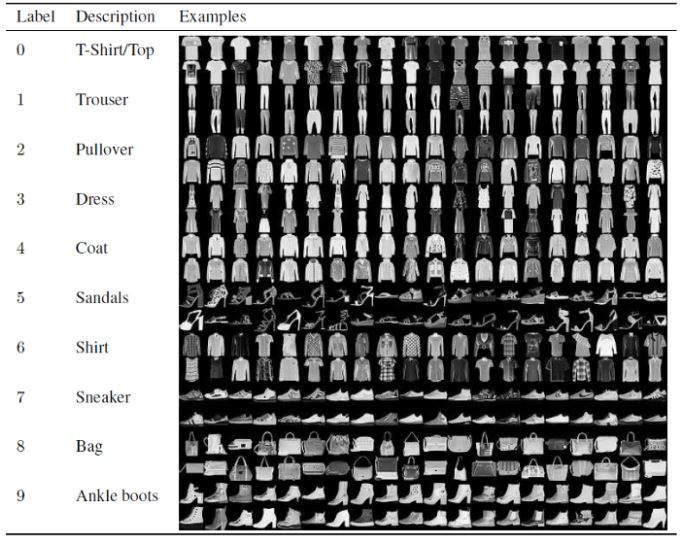Keras has a remarkably powerful Python-based neural network API, and it runs on top of Tensorflow. I encourage you to look at this guide to get familiar with the components of CNN and how it manipulates the images to perform complex computer vision tasks.

Image data is made up of pixels. In black and white images, each pixel displays a number ranging from 0 to 255. A color image contains the pixel combination red (R), green (G), blue (B), each ranging from 0 to 255. If an image has a resolution of 748 x 1005, it is a grid with 748 columns and 1005 rows. So that will be 748*1005 = 0.75 megapixels.

## What are Autoencoders?

Autoencoders are tagged under self-supervised learning. Some say it's unsupervised as they are independent of the labeled responses when coming to classification. They are used by neural networks to perform representation learning. In the image below, the autoencoders contain a bottleneck network that performs compressed knowledge representation for the input. To leverage the autoencoders performing, you need to make sure that they carefully recreate observation and also learn generalized encoding and decoding methods on the training data. In autoencoders, middle layers/hidden core layers are of more value than the output layer.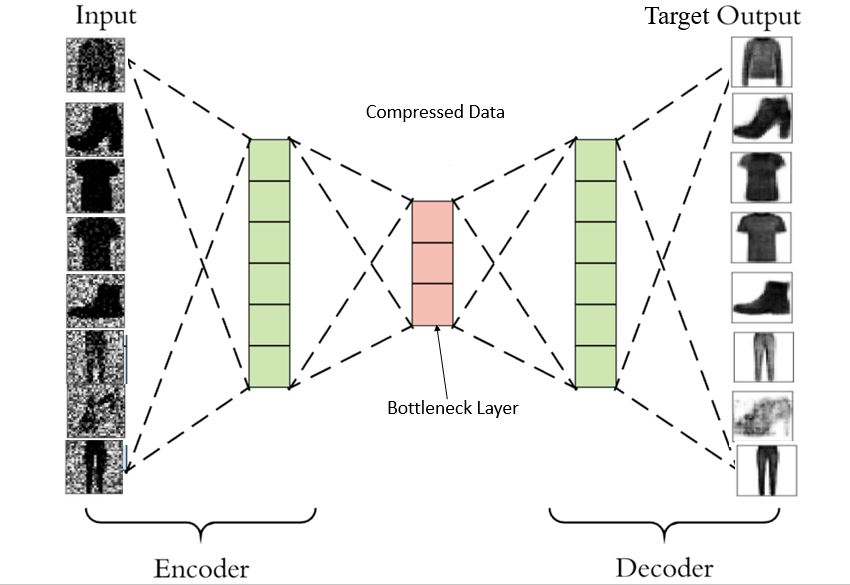If the number of neurons in the middle layer is less than the number of neurons in the input layer, the network extracts the more effective information. The middle layer will not have any other option but to learn the most important image patterns, ignoring the noises. If you have more neurons in the middle layer, the neural network will have a higher capacity to learn the pattern, making the network lazy. It will copy and paste the input values to the output values, learn noises, and not extract any feature.

Hence, the bottleneck model is essential.

The guides Encoders and Decoders for Neural Machine Translation and NMT: Encoder and Decoder with Keras discuss how encoder and decoder models work hand in hand to produce a giant model used for machine translation. Here, in image denoising, the encoding network will compress the input layer values (bottleneck). Its results will work as input to the middle layer. The decoder network's job is to reconstruct the information and provide the results. Most computer vision engineers follow symmetry/mirror arrangement when it comes to the number of hidden layers, meaning that the number of hidden layers and neurons in the encoder network will be the same in the decoder network.

## Why Autoencoders?

To remove the noise from an image, it is important to reduce its dimensionality. Principal Component Analysis (PCA) is used to perform this task. But PCA has limitations; it only applies linear transformation and also contains outliers. On the other hand, autoencoders can introduce non-linearity into the network with the help of their non-linear activation functions and the stack of multiple layers. Outliers, a by-product of dimensionality reduction, can easily be detected by using this neural network.

## Import Libraries and Load Dataset

The example in this guide will take a reference for Keras implementation on Fashion MNIST image modeling. This guide runs on Google Colab GPU. I would strongly recommend using GPU as it improves the training time drastically. Go to Edit > Notebook Settings, make changes, and save.

Skip this part if you are working on a different IDE or aware of how google Colab handles the data.

If you load your data in a normal folder in Colab, it will be temporarily present. Before starting, mount your drive in the Colab.

``````1from google.colab import drive
2drive.mount('/content/drive') ``````
python

Copy and paste the authentication code and press enter.

You are all set! Import the important libraries and modules.

``````1import seaborn as sns
2import numpy as np
3import pandas as pd
4
5from sklearn.model_selection import train_test_split
6
7import matplotlib.pyplot as plt
8from tensorflow.keras.models import Sequential, Model
9from tensorflow.keras.layers import Dense, Input
10from tensorflow.keras.utils import to_categorical
11%matplotlib inline
12sns.set(style = 'white', context = 'notebook', palette = 'deep')
13np.random.seed(42) ``````
python

To read the CSV data, you will need `pandas.read_csv`. It will read the data into the Pandas data frame. Or you can use `keras.dataset` in the library and import `fashion_mnist`. Use `fashion.mnist.load_data()` to use the dataset.

``````1train = pd.read_csv("/content/drive/My Drive/fashion-mnist_test.csv")
python

Check how pixels look like in the data frame. `train.head()` will show the first five columns of the data frame.

``1train.head() ``
python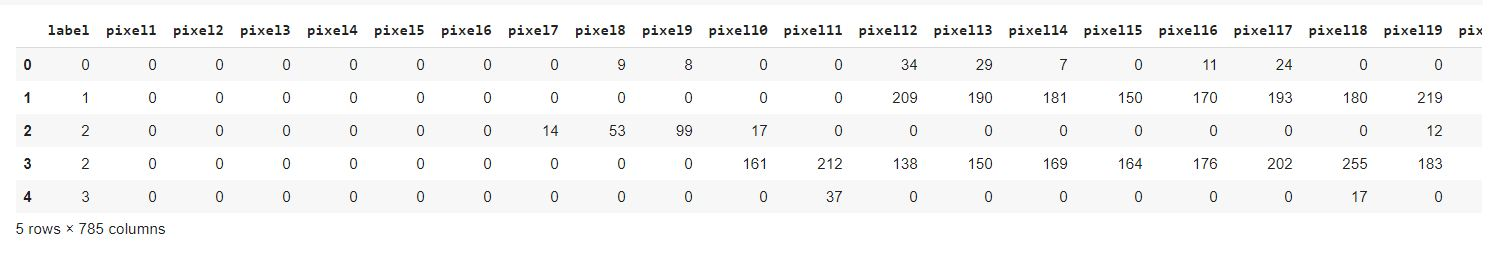So there are 784 total pixels present in the data of size 28x28. A black and white image is in a 2D array form.

## Preparing the Image Data

The data contains black and white images with unsigned integers of the range 0 to 255.

Here there is a need for scaling the image. Normalize the pixel values by rescaling them to the range 0-1. The first step is to convert the data type from the data frame and series to NumPy `ndarray`.

``````1y_train = train["label"]
2x_train = train.drop(labels = ["label"], axis = 1)
3
4print(type(x_train))
5print(type(y_train)) ``````
python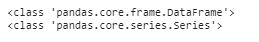``````1x_train = x_train.to_numpy()
2y_train = y_train.to_numpy()
3
4print(type(x_train))
5print(type(y_train)) ``````
python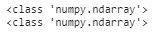``1x_train = x_train.astype('float')/255. ``
python

Now by using the holdout method, split the training and testing data into an 80:20 ratio.

``1x_train, x_val, y_train, y_val = train_test_split(x_train, y_train, test_size = 0.2, random_state = 42) ``
python

Check the number of samples you got.

``````1x_train_size = len(x_train)
2x_val_size = len(x_val)
3
4print(x_train_size)
5print(x_val_size) ``````
python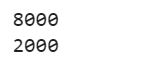To develop a generalized model, a bit of noise is added to the input data to make it corrupt. The uncorrupted data is maintained, and it acts as the output. Here the model cannot memorize the training data and maps out the result as input. Output targets are different. This forces the model to map the input data to a lower-dimension manifold (a concentration point for input data). Consider an example where the data is comprised of car images; all images that look like cars would be part of a manifold. If this manifold is accurately detected then the added noise can be skipped. You can refer to this paper to gain more knowledge.

Add synthetic noise by applying random data on the image data. You will need to normalize that new form of random image too. To achieve that, multiply the random noise by 0.9 and clip the range between 0 to 1. You may also use the Gaussian noise matrix and notice the difference.

``````1#method-1
2x_train_noisy = x_train + np.random.rand(x_train_size, 784) * 0.9
3x_val_noisy = x_val + np.random.rand(x_val_size, 784) * 0.9
4
6# x_train_noisy = x_train + 0.75 * np.random.normal(loc=0.0, scale=1.0, size=x_train.shape)
7# x_val_noisy = x_val + 0.75 * np.random.normal(loc=0.0, scale=1.0, size=x_val.shape)
8
9x_train_noisy = np.clip(x_train_noisy, 0., 1.)
10x_val_noisy = np.clip(x_val_noisy, 0., 1.) ``````
python

Only for the visualization purpose, the image is reshaped from 1D array to 2D array, 784 to (28,28).

``````1def plot(x, p , labels = False):
2 plt.figure(figsize = (20,2))
3 for i in range(10):
4 plt.subplot(1, 10, i+1)
5 plt.imshow(x[i].reshape(28,28), cmap = 'binary')
6 plt.xticks([])
7 plt.yticks([])
8 if labels:
9 plt.xlabel(np.argmax(p[i]))
10 plt.show()
11 return
12plot(x_train, None) ``````
python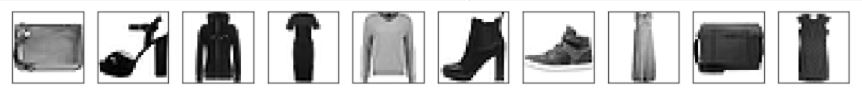``1plot(x_train_noisy,None) ``
python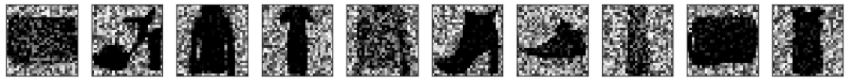The input size is of a 1D array. Notice that Dense layer 64 produces the bottleneck. The final layer at the decoder end gives the output of 784 units. The sigmoid function gives out the value between 0 and 1. This layer decides whether to consider the noise pixel or not.

``````1input_image = Input(shape = (784, ) )
2
3encoded = Dense(512, activation = 'relu')(input_image)
4encoded = Dense(512, activation = 'relu')(encoded)
5encoded = Dense(256, activation = 'relu')(encoded)
6encoded = Dense(256, activation = 'relu')(encoded)
7encoded = Dense(64, activation = 'relu')(encoded)
8
9decoded = Dense(512, activation = 'relu')(encoded)
10decoded = Dense(784, activation = 'sigmoid')(decoded)
11
12autoencoder = Model(input_image, decoded)
13autoencoder.compile(loss= 'binary_crossentropy' , optimizer = 'adam')
14autoencoder.summary() ``````
python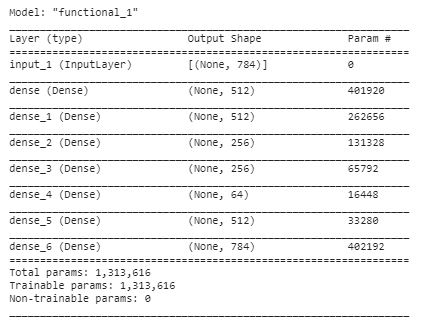The input size is of the 1D array. Notice that the Dense layer `64` produces the bottleneck. The last layer at the decoder end gives the output of 784 units. The sigmoid function gives out the value between 0 and 1. This layer decides if to consider the noise pixel.

``````1import tensorflow as tf
2history = autoencoder.fit(x_train_noisy, x_train, epochs=100, batch_size=128,
3 shuffle = True, validation_data=(x_val_noisy, x_val)) ``````
python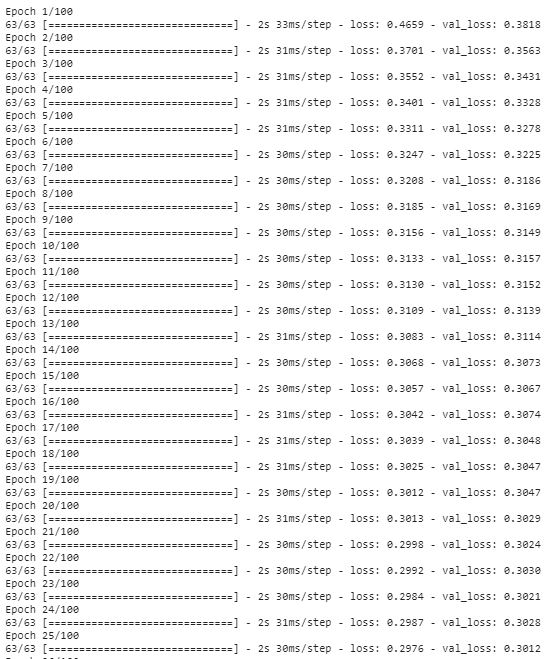## Producing a Denoised Image

Below you can see how well denoised images were produced from noisy ones present in `x_val`. There are three outputs: original test image, noisy test image, and denoised test image form autoencoders.

``1preds = autoencoder.predict(x_val_noisy) ``
python
``````1print("Test Image")
2plot(x_val, None) ``````
python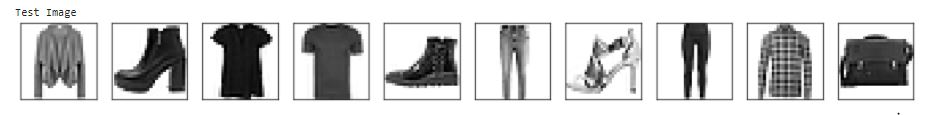``````1print("Noisy Image")
2plot(x_val_noisy, None) ``````
python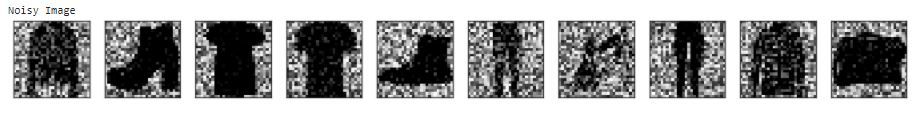``````1print("Denoised Image")
2plot(preds, None)
3 ``````
python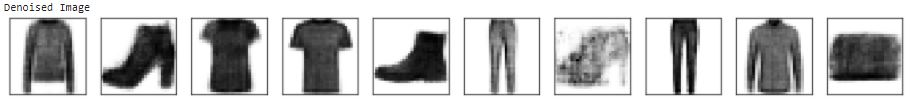Plot the loss.

``````1def plot_loss(history, x = 'loss', y = 'val_loss'):
2 fig, ax = plt.subplots( figsize=(20,10))
3 ax.plot(history.history[x])
4 ax.plot(history.history[y])
5 plt.title('Model Loss')
6 plt.ylabel(y)
7 plt.xlabel(x)
8 plt.legend(['Train', 'Val'], loc='upper left')
9 ax.grid(color='black')
10 plt.show() ``````
python
``1plot_loss(history) ``
python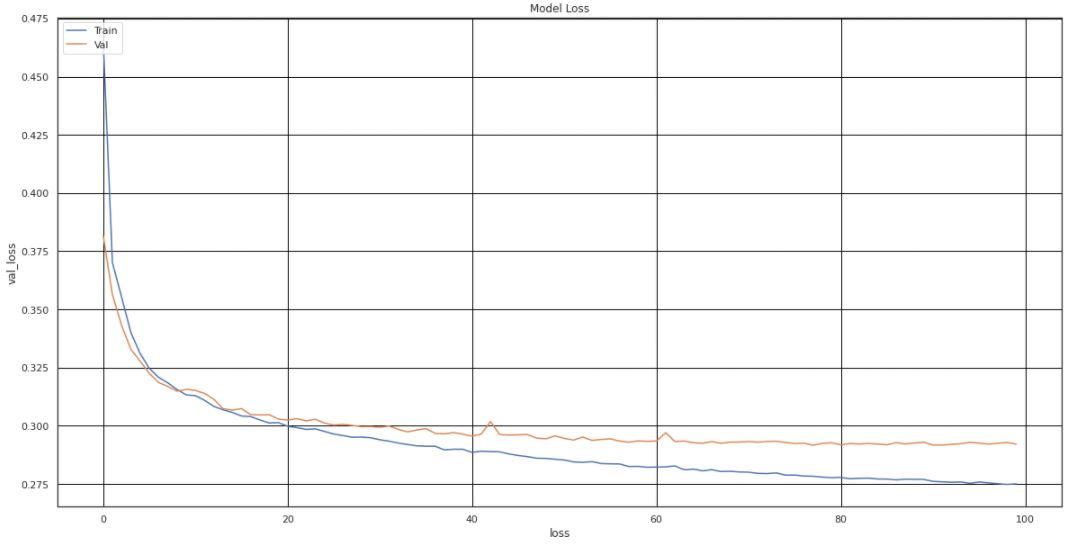## Conclusion

The result is good but there is still scope for narrowing the gap. Play with the hyper-parameters. You can leverage the TensorBoard HParam feature that helps you track the progress and visualize it. Adjust the number of epochs. Increase/decrease the layers in the model check for the results each time.

But what if you have huge image data? The training will take a lot of time. Keras and Pytorch both have many pre-trained CNNs including, ResNet, VGG, DenseNet, and MobileNet. They use a large image database. ImageNet is an open source database you can download for your research and also contribute to.

Further, you may combine the noise reduction model with the classification model. The autoencoders will try to enhance the image. If you have any questions, feel free to reach out to me at CodeAlphabet.